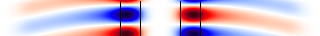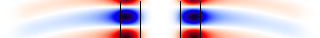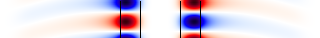# Ring Resonator in Cylindrical Coordinates

In Tutorial/Basics/Modes of a Ring Resonator, we computed the modes of a ring resonator by performing a 2d simulation. Here, we will simulate the same structure while exploiting the fact that the system has continuous rotational symmetry, by performing the simulation in cylindrical coordinates. See also ring-cyl.py.

Table of Contents

## The Python Script

We begin by importing the meep and argparse library modules:

import meep as mp
import argparse

def main(args):


We then define the parameters of the problem with exactly the same values as in the 2d simulation:

n = 3.4     # index of waveguide
w = 1       # width of waveguide
r = 1       # inner radius of ring
pad = 4     # padding between waveguide and edge of PML
dpml = 2    # thickness of PML


Now, we'll define the dimensions and size of the computational cell:

sr = r + w + pad + dpml         # radial size (cell is from 0 to sr)
dimensions = mp.CYLINDRICAL
cell = mp.Vector3(sr, 0, 0)


The key thing is to set the dimensions parameter to CYLINDRICAL. This means that all vectors will represent ($r$,φ,$z$) coordinates instead of ($x$,$y$,$z$). The computational cell in the $r$ direction is of size sr = r + w + pad + dpml, and runs from 0 to sr (by default) rather than from -sr/2 to sr/2 as it would for any other dimension. Note that our $z$ size is 0 because it is in 2d. The φ size is also 0, corresponding to the continuous rotational symmetry. A finite φ size might correspond to discrete rotational symmetry, but this is not currently supported.

In particular, in systems with continuous rotational symmetry, by an analogue of Bloch's theorem, the angular dependence of the fields can always be chosen in the form $\exp(i m φ)$ for some integer $m$. Meep uses this fact to treat the angular dependence analytically, with $m$ given by the input variable m which we'll set to a command-line argument that is 3 by default.

m = args.m


Thus, we are essentially performing a 1d calculation, where Meep must discretize the $r$ direction only. For this reason, it will be much faster than the previous 2d calculation.

The geometry is now specified by a single Block object — remember that this is a block in cylindrical coordinates, so that it really specifies an annular ring:

geometry = [mp.Block(center=mp.Vector3(r + (w / 2)),
size=mp.Vector3(w, 1e20, 1e20),
material=mp.Medium(index=n))]

pml_layers = [mp.PML(dpml)]
resolution = 10


We have added PML layers on "all" sides. Meep, however, notices that the $z$ direction has no thickness and automatically makes it periodic with no PML. Meep also omits PML from the boundary at $r$=0 which is handled by the analytical reflection symmetry.

Now, the remaining inputs are almost exactly the same as in the previous 2d simulation. We'll add a single Gaussian point source in the $z$ direction to excite $E_z$-polarized modes, with some center frequency and width:

fcen = args.fcen  # pulse center frequency
df = args.df      # pulse width (in frequency)
sources = [mp.Source(src=mp.GaussianSource(fcen, fwidth=df),
component=mp.Ez,
center=mp.Vector3(r + 0.1))]


Note that this isn't really a point source, however, because of the cylindrical symmetry — it is really a ring source with φ dependence $\exp(i m \phi)$. Finally, as before, we run until the source has turned off, plus 200 additional time units during which we use Harminv to analyze the $E_z$ field at a given point to extract the frequencies and decay rates of the modes.

sim = mp.Simulation(cell_size=cell,
geometry=geometry,
boundary_layers=pml_layers,
resolution=resolution,
sources=sources,
dimensions=dimensions,
m=m)

sim.run(mp.after_sources(mp.Harminv(mp.Ez, mp.Vector3(r + 0.1), fcen, df)),
until_after_sources=200)


At the very end, we'll also output one period of the fields to make movies, etcetera. A single field output would be a 1d dataset along the $r$ direction, so to make things more interesting we'll use to_appended to append these datasets to a single HDF5 file to get an $r \times t$ 2d dataset. We'll also use in_volume to specify a larger output volume than just the computational cell: in particular, we'll output from -sr to sr in the $r$ direction, where Meep will automatically infer the $-r$ field values from the reflection symmetry.

sim.run(mp.in_volume(mp.Volume(center=mp.Vector3(), size=mp.Vector3(2 * sr)),
mp.at_beginning(mp.output_epsilon),
mp.to_appended("ez", mp.at_every(1 / fcen / 20, mp.output_efield_z))),
until=1 / fcen)


The last component of the script invovles defining the three command-line arguments and their default values:

if __name__ == '__main__':
parser = argparse.ArgumentParser()
parser.add_argument('-fcen', type=float, default=0.15, help='pulse center frequency')
parser.add_argument('-df', type=float, default=0.1, help='pulse frequency width')
parser.add_argument('-m', type=int, default=3, help='phi (angular) dependence of the fields given by exp(i m phi)')
args = parser.parse_args()
main(args)


## Results

Now, we are ready to run our simulation. Recall that, in the 2d calculation, we got three modes in this frequency range: one at ω=0.11785 with $Q$=77 and an $m$=3 field pattern, one at ω=0.14687 with $Q$=351 and an $m$=4 field pattern, and one at ω=0.17501 with $Q$=1630 and an $m$=5 field pattern. We should get the same modes here with some differences due to the finite resolution, except now that we will have to run three calculations, a separate one for each value of $m$. It will still be much faster than before because the simulations are 1d instead of 2d.

In particular, we'll run:

unix% python ring-cyl.py -m 3 | grep harminv
unix% python ring-cyl.py -m 4 | grep harminv
unix% python ring-cyl.py -m 5 | grep harminv


giving the combined output:

harminv0:, frequency, imag. freq., Q, |amp|, amplitude, error
harminv0:, 0.11835455441250631, -0.0006907792691647415, 85.66741917111612, 0.02570190626349302, (-0.02402703883357199-0.00912630212448642j), (5.286949731053267e-10+0j)
harminv0:, 0.1475578747705309, -0.0001938438860632441, 380.61008208014414, 0.19361245519715206, (0.1447225471614173+0.12861246887677943j), (5.889273063545974e-11+0j)
harminv0:, 0.1759448592380757, -4.900590034953583e-05, 1795.1395442502285, 0.0452479314013276, (-0.014395016792255884-0.042897072017212545j), (1.6343462235932872e-10+0j)


This is indeed very close to the 2d simulations: the frequencies are within 1% of the previous values. The $Q$ values (lifetimes) differ by a larger amount although they are still reasonably close.

Which is more accurate, the 2d or the cylindrical simulation? We can answer this question by increasing the resolutions in both cases and seeing what they converge towards. In particular, let's focus on the $m$=4 mode. In the cylindrical case, if we double the resolution to 20 we get ω=0.14748 and $Q$=384. In the 2d case, if we double the resolution to 20 we get ω=0.14733 and $Q$=321. So, it looks like the frequencies are clearly converging together and that the cylindrical simulation is more accurate (as you might expect since it describes the φ direction analytically). But the $Q$ values seem to be getting farther apart — what's going on?

The problem is twofold. First, there is some signal-processing error in determining $Q$ in the 2d case, as indicated by the "error" column of the harminv output which is only 4e-7 for the 2d simulation vs. 6e-11 for the cylindrical case. We can bring this error down by running with a narrower bandwidth source, which excites just one mode and gives a cleaner signal, or by analyzing over a longer time than 200. Doing the former, we find that the 2d value of $Q$ at a resolution of 20 should really be $Q$=343. Second, PML absorbing layers are really designed to absorb planewaves incident on flat interfaces, but here we have a cylindrical PML layer. Because of this, there are larger numerical reflections from the PML in the cylindrical simulation, which we can rectify by pushing the PML out to a larger radius (i.e. using a larger value of pad) and/or increasing the PML thickness (increasing dpml) so that it turns on more adiabatically. In the cylindrical simulation for resolution = 20, if we increase to dpml = 16, we get $Q$=343, which is in much better agreement with the 2d calculation and if we increase to dpml = 32 we get the same $Q$=343, so it seems to be converged.

This illustrates the general principle that you need to check several parameters to ensure that results are converged in time-domain simulations: the resolution, the run time, the PML thickness, etcetera.

Finally, we can get the field images. Since we only are exciting one mode per m here anyway, according to harminv, we don't really need to use a narrow-band source. We'll do so anyway just to remind you of the general procedure, however, e.g. for the ω=0.118, $m$=3 mode:

unix% python ring-cyl.py -m 3 -fcen 0.118 -df 0.01
unix% h5topng -S 2 -Zc dkbluered -C ring-cyl-eps-001200.00.h5 ring-cyl-ez.h5


Note that, because of the to_appended command, the ring-cyl-ez.h5 file is a 160$\times$18 dataset corresponding to an $r \times t$ slice. Repeating this for all three modes results in the images:

$E_z$ for ω=0.118 $m$=3 mode:$E_z$ for ω=0.148 $m$=4 mode:$E_z$ for ω=0.176 $m$=5 mode:Because we are looking only at a φ=0 slice, the visual distinction between $m$ values is much less than with the 2d simulation. What is apparent is that, as the frequency increases, the mode becomes more localized in the waveguide and the radiating field (seen in the $r \times t$ slice as curved waves extending outward) becomes less, as expected.